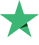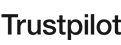смотрят
4 студентов
89 Количество лекций
Средний

#### Что вы изучите?

• Learn Complete Supervised Machine Learning Models in Python
• Learn the Math behind every Machine Learning Model
• Learn the Intuition of each Model
• Learn to make simple and GUI Based Templates
• Learn to choose the best Machine Learning Model for a specific problem

#### Содержание

11ч 17м
10:17
##### Раздел 2: Simple Linear Regression Intuition
36:13
Simple Linear Regression Intuition 3
20:40
##### Раздел 3: Simple Linear Regression in Python
1:00:41
Simple Linear Regression in Python 1
13:01
Simple Linear Regression in Python 2
06:37
Simple Linear Regression in Python 3
05:13
Simple Linear Regression in Python 4
05:53
Simple Linear Regression in Python 5
05:04
Simple Linear Regression in Python 6
06:32
Simple Linear Regression in Python 7
18:21
##### Раздел 4: Multiple Linear Regression Intuition
55:39
Multiple Linear Regression Intuition 1
09:22
Multiple Linear Regression Intuition 2
05:51
Multiple Linear Regression Intuition 3
10:38
Multiple Linear Regression Intuition 4
13:30
Multiple Linear Regression Intuition 5
07:21
Multiple Linear Regression Intuition 6
08:57
##### Раздел 5: Multiple Linear Regression in Python
1:00:53
Multiple Linear Regression in Python 1
07:18
Multiple Linear Regression in Python 2
07:30
Multiple Linear Regression in Python 3
10:16
Multiple Linear Regression in Python 4
09:21
Multiple Linear Regression in Python 5
09:44
Multiple Linear Regression in Python 6
06:58
Multiple Linear Regression in Python 7
09:46
##### Раздел 6: Polynomial Regression Intuition
07:12
Polynomial Regression Intuition 1
07:12
##### Раздел 7: Polynomial Regression in Python
24:54
Polynomial Regression in Python 1
04:06
Polynomial Regression in Python 2
09:36
Polynomial Regression in Python 3
11:12
##### Раздел 8: Ridge Regression Intuition
14:54
Ridge Regression Intuition 1
03:37
Ridge Regression Intuition 2
11:17
##### Раздел 9: Ridge Regression in Python
32:17
Ridge Regression in Python 1
11:57
Ridge Regression in Python 2
10:28
Ridge Regression in Python 3
05:19
Ridge Regression in Python 4
04:33
##### Раздел 10: Lasso Regression Intuition
08:57
Lasso Regression Intuition 1
08:57
##### Раздел 11: Lasso Regression in Python
28:59
Lasso Regression in Python 1
13:53
Lasso Regression in Python 2
07:33
Lasso Regression in Python 3
05:31
Lasso Regression in Python 4
02:02
##### Раздел 12: Decision Tree Regression Intuition
06:54
Decision Tree Regression Intuition 1
06:54
##### Раздел 13: Decision Tree Regression in Python
44:47
Decision Tree Regression in Python 1
07:42
Decision Tree Regression in Python 2
10:56
Decision Tree Regression in Python 3
07:46
Decision Tree Regression in Python 4
09:52
Decision Tree Regression in Python 5
08:31
##### Раздел 14: Random Forest Regression Intuition
09:47
Random Forest Regression Intuition 1
09:47
##### Раздел 15: Random Forest Regression in Python
21:42
Random Forest Regression in Python 1
06:37
Random Forest Regression in Python 2
07:31
Random Forest Regression in Python 3
07:34
##### Раздел 16: ++++++++++++++Beginning Classifications++++++++++++++
12:06
Confusion Matrix
07:05
Sparse Matrix
03:37
Data set for Classification Models
01:24
06:05
KNN Intuition 1
06:05
24:24
KNN in Python 1
14:06
KNN in Python 2
10:18
##### Раздел 19: Logistic Regression Intuition
12:23
Logistic Regression Intuition
12:23
##### Раздел 20: Logistic Regression in Python
30:02
Logistic Regression Python 1
05:33
Logistic Regression Python 2
04:07
Logistic Regression Python 3
03:22
Logistic Regression Python 4
05:51
Logistic Regression Python 5
07:02
Logistic Regression Python 6
04:07
##### Раздел 21: Decision Tree Classification Intuition
20:06
Decision Tree Classification Intuition 1
08:23
Decision Tree Classification Intuition 2
11:43
##### Раздел 22: Decision Tree Classification in Python
20:00
Decision Tree Classification in Python 1
05:36
Decision Tree Classification in Python 2
06:30
Decision Tree Classification in Python 3
05:29
Decision Tree Classification in Python 4
02:25
##### Раздел 23: Random Forest Classification Intuition
10:40
Random Forest Classification Intuition
10:40
##### Раздел 24: Random Forest Classification in Python
32:10
Random Forest Classification in Python 1
07:09
Random Forest Classification in Python 2
03:07
Random Forest Classification in Python 3
11:35
Random Forest Classification in Python 4
08:02
Random Forest Classification in Python 5
02:17
##### Раздел 25: Naiive Bayes Classification Intuition
19:00
Naive Bayes Intuition 1
08:52
Naive Bayes Intuition 2
10:08
##### Раздел 26: Naiive Bayes Classification in Python
29:00
Naiive Bayes Classification in Python 1
07:19
Naiive Bayes Classification in Python 2
03:01
Naiive Bayes Classification in Python 3
09:36
Naiive Bayes Classification in Python 4
09:04
##### Раздел 27: Support Vector Classification Intuition
08:12
Support Vector Classification Intuition 1
08:12
##### Раздел 28: Support Vector Classification in Python
29:43
Support Vector Classification in Python 1
04:56
Support Vector Classification in Python 2
03:27
Support Vector Classification in Python 3
09:28
Support Vector Classification in Python 4
09:31
Support Vector Classification in Python 5
02:21

#### Требования

• Basics of Python

Отзывы 0
Студенты 0
Курсы 6

#### Отзывы о платформе BitDegree

Наша оценка - Отлично
9.5 из 10